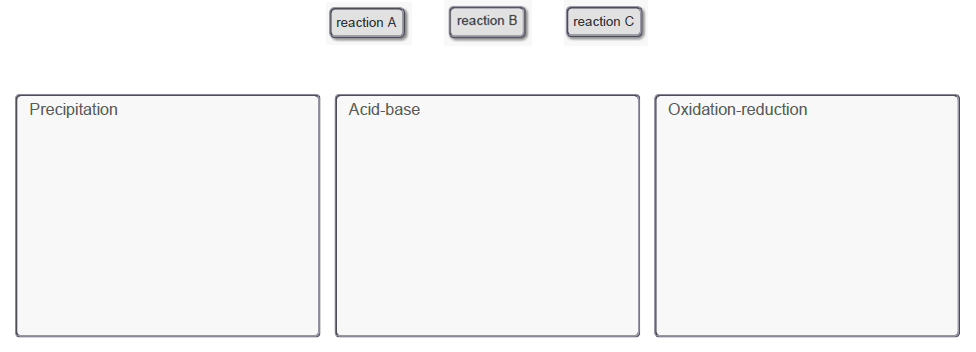# Problem: Classify the following reactions:A. HCl(aq) + NaOH(aq) → NaCl(aq) + H2O(l)B. Ba(OH)2(aq) + ZnCl2(aq) → BaCl2(aq) + Zn(OH)2(s)C. 2 AgNO3(aq) + Mg(s) → Mg(NO3)2(aq) + 2 Ag(s)Drag the appropriate items to the ir respective bins.The laundry detergent and bath salt reaction is an example of a precipitation reaction in which two aqueous ionic substances react to form an insoluble ionic substance called a precipitate. Acid-base reactions involve an acid and a base reacting to form a salt. Oxidation-reduction reactions involve the transfer of electrons, which you can recognize by changes in oxidation number.

###### FREE Expert SolutionView Complete Written Solution
###### Problem Details

Classify the following reactions:

A. HCl(aq) + NaOH(aq) → NaCl(aq) + H2O(l)
B. Ba(OH)2(aq) + ZnCl2(aq) → BaCl2(aq) + Zn(OH)2(s)
C. 2 AgNO3(aq) + Mg(s) → Mg(NO3)2(aq) + 2 Ag(s)

Drag the appropriate items to the ir respective bins.The laundry detergent and bath salt reaction is an example of a precipitation reaction in which two aqueous ionic substances react to form an insoluble ionic substance called a precipitate. Acid-base reactions involve an acid and a base reacting to form a salt. Oxidation-reduction reactions involve the transfer of electrons, which you can recognize by changes in oxidation number.